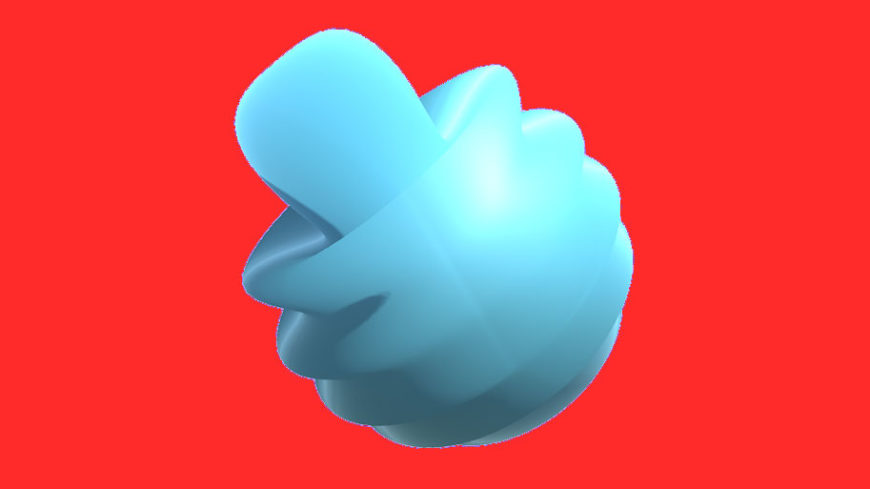# Jelly Shader, Part 7: Project Clean-Up and Final StepsPart 7 – Project Clean-Up and Final Steps
This is article 7 in our multi-part series on developing a custom shader for Unity 3D. In the final article in this series we will focus on last touch-ups that will make this shader usable in your next project.
Our project is now mostly complete, but I’d like to add one more variable to the shader and make our shader values configurable from inside the editor. First, inside the subshader, we’re going to modify all our static variables to be non-static. We’re also going to add a new variable to the bottom of the list called `_WaveSpeed`. That section of your subshader should look like this.``` half _Frequency; half _Amplitude; half _WaveFalloff; half _MaxWaveDistortion; half _ImpactSpeed; half _WaveSpeed; ```

The Mysterious Disappearing Assignment

You may have noticed that we also removed the assignment operation from our declaration. Now that these variables are no longer marked as static, we can’t assign them in their declaration. They will be overwritten when the shader is initialized. This is fine though because we will be passing in values for these variables from our UI.

We also need to expose all these variables to the editor, to do that, add them to the properties section of our shader.
``` _Frequency ("Frequency", Range(0, 1000)) = 10 _Amplitude ("Amplitude", Range(0, 5)) = 0.2 _WaveFalloff ("Wave Falloff", Range(1, 8)) = 4 _MaxWaveDistortion ("Max Wave Distortion", Range(0.1, 2.0)) = 1 _ImpactSpeed ("Impact Speed", Range(0, 10)) = 0.5 _WaveSpeed ("Wave Speed", Range(-10, 10)) = -5 ```

Next we need to actually include `_WaveSpeed` in our vertex calculation. Add it here.

``` v.vertex.xyz += v.normal * sin(impactAxis * _Frequency + _ControlTime * _WaveSpeed) * _Amplitude * (1 / dist); ```
Fantastic! Our shader is now complete and all of our configuration values are editable from the inspector. I’ve tried to include some sensible defaults for an interesting jelly effect but feel free to play with the values and find your own interesting effects. To find those values in the editor, select the sphere inside the Unity editor and expand the JellyMaterial component near the bottom of the Inspector. The final shader and script code is pasted below for your convenience.

`JellyShader.shader`
```Shader "Custom/JellyShader" { Properties { _Color ("Color", Color) = (1,1,1,1) _MainTex ("Albedo (RGB)", 2D) = "white" {} _Glossiness ("Smoothness", Range(0,1)) = 0.5 _Metallic ("Metallic", Range(0,1)) = 0.0```

```_ControlTime ("Time", float) = 0 _ModelOrigin ("Model Origin", Vector) = (0,0,0,0) _ImpactOrigin ("Impact Origin", Vector) = (-5,0,0,0)```

```_Frequency ("Frequency", Range(0, 1000)) = 10 _Amplitude ("Amplitude", Range(0, 5)) = 0.1 _WaveFalloff ("Wave Falloff", Range(1, 8)) = 4 _MaxWaveDistortion ("Max Wave Distortion", Range(0.1, 2.0)) = 1 _ImpactSpeed ("Impact Speed", Range(0, 10)) = 0.5 _WaveSpeed ("Wave Speed", Range(-10, 10)) = -5 }```
```SubShader { Tags { "RenderType"="Opaque" } LOD 200```

```CGPROGRAM // Physically based Standard lighting model, and enable shadows on all light types #pragma surface surf Standard fullforwardshadows addshadow vertex:vert```

```// Use shader model 3.0 target, to get nicer looking lighting #pragma target 5.0```

`sampler2D _MainTex;`

```struct Input { float2 uv_MainTex; };```

```half _Glossiness; half _Metallic; fixed4 _Color;```

```float _ControlTime; float4 _ModelOrigin; float4 _ImpactOrigin;```

```half _Frequency; //Base frequency for our waves. half _Amplitude; //Base amplitude for our waves. half _WaveFalloff; //How quickly our distortion should fall off given distance. half _MaxWaveDistortion; //Smaller number here will lead to larger distortion as the vertex approaches origin. half _ImpactSpeed; //How quickly our wave origin moves across the sphere. half _WaveSpeed; //Oscillation speed of an individual wave.```

```// Add instancing support for this shader. You need to check 'Enable Instancing' on materials that use the shader. // See https://docs.unity3d.com/Manual/GPUInstancing.html for more information about instancing. // #pragma instancing_options assumeuniformscaling UNITY_INSTANCING_CBUFFER_START(Props) // put more per-instance properties here UNITY_INSTANCING_CBUFFER_END```

```void vert (inout appdata_base v) { float4 world_space_vertex = mul(unity_ObjectToWorld, v.vertex);```

```float4 direction = normalize(_ModelOrigin - _ImpactOrigin); float4 origin = _ImpactOrigin + _ControlTime * _ImpactSpeed * direction;```

```//Get the distance in world space from our vertex to the wave origin. float dist = distance(world_space_vertex, origin);```

```//Adjust our distance to be non-linear. dist = pow(dist, _WaveFalloff);```

```//Set the max amount a wave can be distorted based on distance. dist = max(dist, _MaxWaveDistortion);```

```//Convert direction and _ImpactOrigin to model space for later trig magic. float4 l_ImpactOrigin = mul(unity_WorldToObject, _ImpactOrigin); float4 l_direction = mul(unity_WorldToObject, direction);```

```//Magic float impactAxis = l_ImpactOrigin + dot((v.vertex - l_ImpactOrigin), l_direction);```

```/v.vertex.xyz += v.normal * sin(impactAxis * _Frequency + _ControlTime * _WaveSpeed) * _Amplitude * (1 / dist); }```

```void surf (Input IN, inout SurfaceOutputStandard o) { // Albedo comes from a texture tinted by color fixed4 c = tex2D (_MainTex, IN.uv_MainTex) * _Color; o.Albedo = c.rgb; // Metallic and smoothness come from slider variables o.Metallic = _Metallic; o.Smoothness = _Glossiness; o.Alpha = c.a; } ENDCG } FallBack "Diffuse" } ```

`JellyClickReceiver.cs`
```using System.Collections; using System.Collections.Generic; using UnityEngine;```

`public class JellyClickReceiver : MonoBehaviour {`

```RaycastHit hit; Ray clickRay;```

```Renderer modelRenderer; float controlTime;```

```// Use this for initialization void Start () { modelRenderer = GetComponent(); }```

```// Update is called once per frame void Update () { controlTime += Time.deltaTime;```

```if (Input.GetMouseButtonDown(0)) { clickRay = Camera.main.ScreenPointToRay(Input.mousePosition);```

```if (Physics.Raycast(clickRay, out hit)) { controlTime = 0;```

```modelRenderer.material.SetVector("_ModelOrigin", transform.position); modelRenderer.material.SetVector("_ImpactOrigin", hit.point); } }```

```modelRenderer.material.SetFloat("_ControlTime", controlTime); } }```